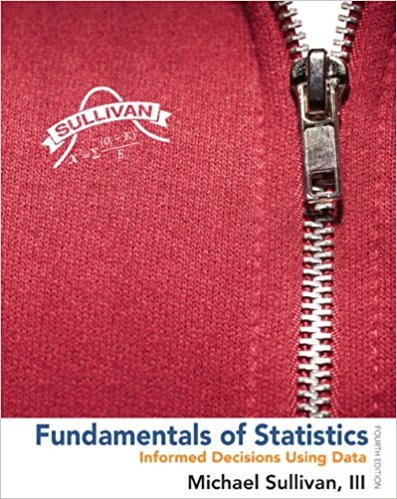×
×

# Solutions for Chapter Chapter 6.1: Fundamentals of Statistics 4th Edition## Full solutions for Fundamentals of Statistics | 4th Edition

ISBN: 9780321838704Solutions for Chapter Chapter 6.1

Solutions for Chapter Chapter 6.1
4 5 0 308 Reviews
23
2
##### ISBN: 9780321838704

This expansive textbook survival guide covers the following chapters and their solutions. Chapter Chapter 6.1 includes 37 full step-by-step solutions. Fundamentals of Statistics was written by and is associated to the ISBN: 9780321838704. This textbook survival guide was created for the textbook: Fundamentals of Statistics, edition: 4. Since 37 problems in chapter Chapter 6.1 have been answered, more than 306852 students have viewed full step-by-step solutions from this chapter.

Key Statistics Terms and definitions covered in this textbook
• Analytic study

A study in which a sample from a population is used to make inference to a future population. Stability needs to be assumed. See Enumerative study

• Assignable cause

The portion of the variability in a set of observations that can be traced to speciic causes, such as operators, materials, or equipment. Also called a special cause.

• Bayes’ estimator

An estimator for a parameter obtained from a Bayesian method that uses a prior distribution for the parameter along with the conditional distribution of the data given the parameter to obtain the posterior distribution of the parameter. The estimator is obtained from the posterior distribution.

• Bivariate normal distribution

The joint distribution of two normal random variables

• Categorical data

Data consisting of counts or observations that can be classiied into categories. The categories may be descriptive.

• Center line

A horizontal line on a control chart at the value that estimates the mean of the statistic plotted on the chart. See Control chart.

• Conidence coeficient

The probability 1?a associated with a conidence interval expressing the probability that the stated interval will contain the true parameter value.

• Conidence level

Another term for the conidence coeficient.

• Continuous random variable.

A random variable with an interval (either inite or ininite) of real numbers for its range.

• Convolution

A method to derive the probability density function of the sum of two independent random variables from an integral (or sum) of probability density (or mass) functions.

• Correlation coeficient

A dimensionless measure of the linear association between two variables, usually lying in the interval from ?1 to +1, with zero indicating the absence of correlation (but not necessarily the independence of the two variables).

• Discrete distribution

A probability distribution for a discrete random variable

• Discrete uniform random variable

A discrete random variable with a inite range and constant probability mass function.

• Error sum of squares

In analysis of variance, this is the portion of total variability that is due to the random component in the data. It is usually based on replication of observations at certain treatment combinations in the experiment. It is sometimes called the residual sum of squares, although this is really a better term to use only when the sum of squares is based on the remnants of a model-itting process and not on replication.

• Error variance

The variance of an error term or component in a model.

• Exhaustive

A property of a collection of events that indicates that their union equals the sample space.

• Forward selection

A method of variable selection in regression, where variables are inserted one at a time into the model until no other variables that contribute signiicantly to the model can be found.

• Fractional factorial experiment

A type of factorial experiment in which not all possible treatment combinations are run. This is usually done to reduce the size of an experiment with several factors.

• Generating function

A function that is used to determine properties of the probability distribution of a random variable. See Moment-generating function

• Goodness of fit

In general, the agreement of a set of observed values and a set of theoretical values that depend on some hypothesis. The term is often used in itting a theoretical distribution to a set of observations.

×